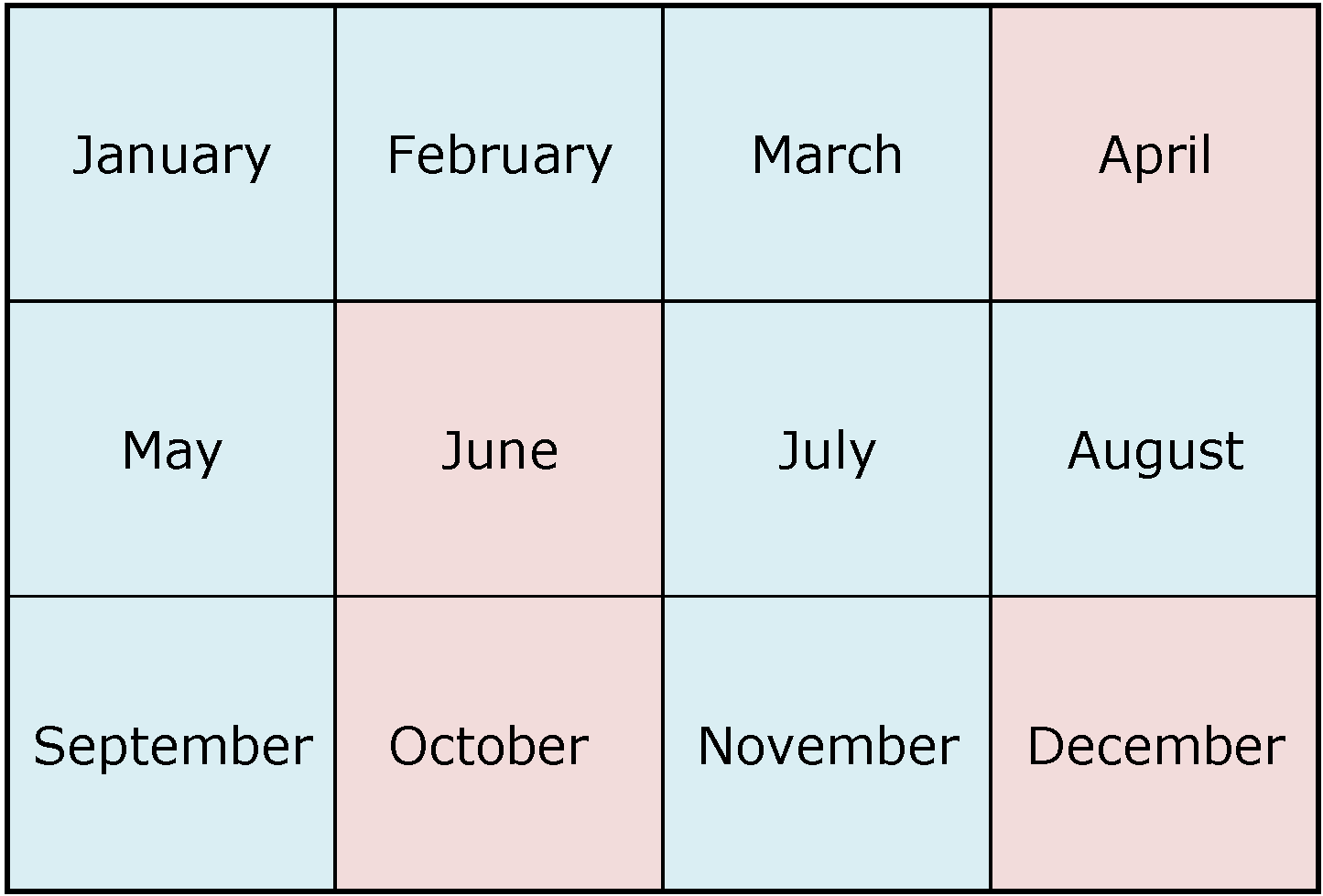ISSN 2284-7995, ISSN Online 2285-3952

## FRACTAL ANALYSIS IN ESTIMATING THE FRAGMENTATION DEGREE OF AGRICULTURAL LANDS

###### Published in Scientific Papers. Series "Management, Economic Engineering in Agriculture and rural development", Vol. 20 ISSUE 3 Written by Florin SALA, Cosmin Alin POPESCU, Mihai Valentin HERBEI

Fractal analysis was used to evaluate the degree of agricultural lands fragmentation. An area in the Western Plain, Romania was studied. The image was taken with the RapidEye satellite system. From the basic image, 10 polygons with equal resolution of 735 x 840 pixels were selected. For each studied polygon, the total surface (TS), the number of plots (PN), the average plot area (APA), and the fractal dimension (D) were determined. Fractal analysis was performed using the box counting method. The correlation analysis revealed a moderate, negative, correlation between PN and APA (r=-0.776), strong negative correlation between D and PN (r=-0.871), respectively a very strong, positive, correlation between D and APA (r=0.935). APA variation according to PN was most faithfully described by a smoothing spline model. Variation of fractal dimension D according to PN was described by a polynomial equation of degree 2, in conditions of R2=0.946, p<< 0.01, and the variation of D according to the APA was described by a polynomial equation of degree 2 in conditions of R2=0.939, p<< 0.01. Based on fractal dimension (D), regression analysis made it possible to estimate PN under conditions of R2=0.818, p=0.0025, F=15.782, respectively APA variation under conditions of R2=0.984, p<< 0.001, F=214.86. Based on PCA, PC1 explained 89.441% of variance, and PC2 explained 10.559% of variance. Cluster analysis led to the grouping of the studied cases, in condition of Coph.corr=0.988.### Find ArticleNext Issue will be published in AprilFour issues are published every year.

The publisher is not responsible for the opinions published in the Volume. They represent the authors’ point of view.

© 2019 To be cited: Scientific Papers. Series “Management, Economic Engineering in Agriculture and Rural Development“.